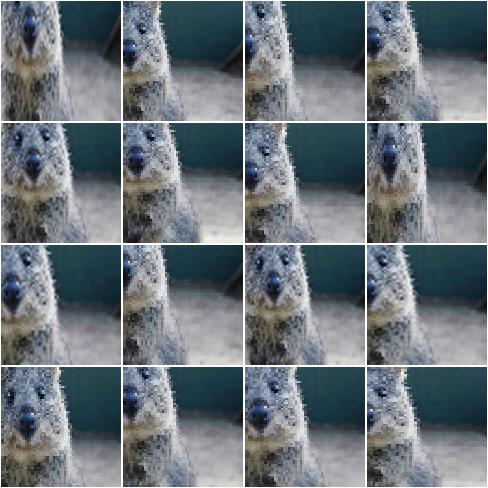# augmenters.size¶

## Resize¶

Augmenter that resizes images to specified heights and widths.

API link: `Resize`

Example. Resize each image to height=32 and width=64:

```import imgaug.augmenters as iaa
aug = iaa.Resize({"height": 32, "width": 64})
```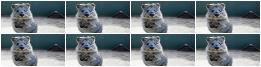Example. Resize each image to height=32 and keep the aspect ratio for width the same:

```aug = iaa.Resize({"height": 32, "width": "keep-aspect-ratio"})
```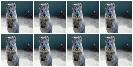Example. Resize each image to something between 50 and 100% of its original size:

```aug = iaa.Resize((0.5, 1.0))
```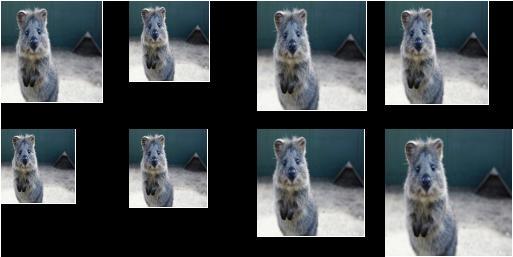Example. Resize each image’s height to 50-75% of its original size and width to either 16px or 32px or 64px:

```aug = iaa.Resize({"height": (0.5, 0.75), "width": [16, 32, 64]})
```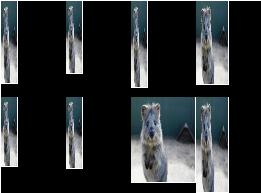Crop/pad images by pixel amounts or fractions of image sizes.

Cropping removes pixels at the sides (i.e. extracts a subimage from a given full image). Padding adds pixels to the sides (e.g. black pixels).

Note

This augmenter automatically resizes images back to their original size after it has augmented them. To deactivate this, add the parameter `keep_size=False`.

API link: `CropAndPad`

Example. Crop or pad each side by up to 10 percent relative to its original size (negative values result in cropping, positive in padding):

```import imgaug.augmenters as iaa
```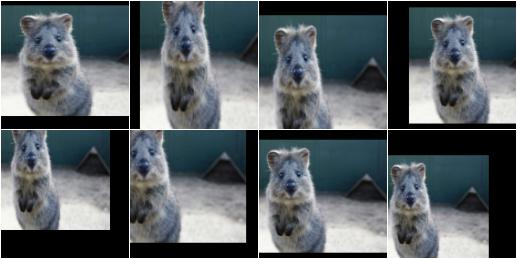Example. Pad each side by 0 to 20 percent. This adds new pixels to the sides. These pixels will either be filled with a constant value (mode=constant) or filled with the value on the closest edge (mode=edge). If a constant value is used, it will be a random value between 0 and 128 (sampled per image).

```aug = iaa.CropAndPad(
percent=(0, 0.2),
)
```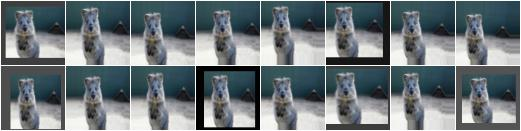Example. Pad the top side of each image by 0 to 30 pixels, the right side by 0-10px, bottom side by 0-30px and left side by 0-10px. Use any of the available modes to fill new pixels and if the mode is constant then use a constant value between 0 and 128.

```aug = iaa.CropAndPad(
px=((0, 30), (0, 10), (0, 30), (0, 10)),
)
```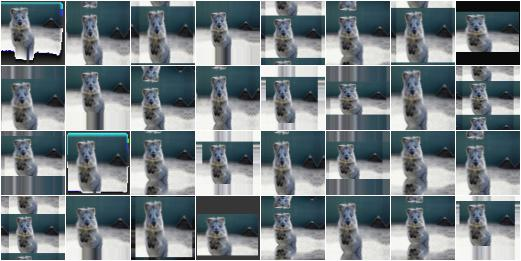Example. Crop/pad each side by up to 10px. The value will be sampled once per image and used for all sides (i.e. all sides gain/lose the same number of rows/colums).

```aug = iaa.CropAndPad(
px=(-10, 10),
sample_independently=False
)
```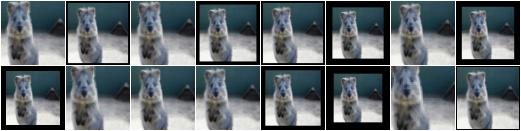This is a shortcut for `CropAndPad`. It only accepts positive pixel/percent values.

API link: `Pad`

## Crop¶

Crop images, i.e. remove columns/rows of pixels at the sides of images.

This is a shortcut for `CropAndPad`. It only accepts positive pixel/percent values and transfers them as negative values to `CropAndPad`.

API link: `Crop`

If images are already at the minimum width/height or are larger, they will not be padded. Note that this also means that images will not be cropped if they exceed the required width/height.

The augmenter randomly decides per image how to distribute the required padding amounts over the image axis. E.g. if 2px have to be padded on the left or right to reach the required width, the augmenter will sometimes add 2px to the left and 0px to the right, sometimes add 2px to the right and 0px to the left and sometimes add 1px to both sides. Set position to `center` to prevent that.

API link: `PadToFixedSize`

Example. For image sides smaller than `100` pixels, pad to `100` pixels. Do nothing for the other edges. The padding is randomly (uniformly) distributed over the sides, so that e.g. sometimes most of the required padding is applied to the left, sometimes to the right (analogous top/bottom). The input image here has a size of `80x80`.

```import imgaug.augmenters as iaa
```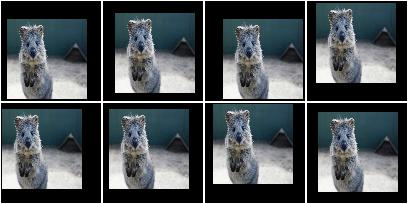Example. For image sides smaller than `100` pixels, pad to `100` pixels. Do nothing for the other image sides. The padding is always equally distributed over the left/right and top/bottom sides. The input image here has a size of `80x80`.

```aug = iaa.PadToFixedSize(width=100, height=100, position="center")
```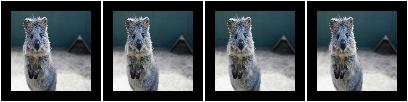Example. For image sides smaller than `100` pixels, pad to `100` pixels and use any possible padding mode for that. Do nothing for the other image sides. The padding is always equally distributed over the left/right and top/bottom sides. The input image here has a size of `80x80`.

```aug = iaa.PadToFixedSize(width=100, height=100, pad_mode=ia.ALL)
```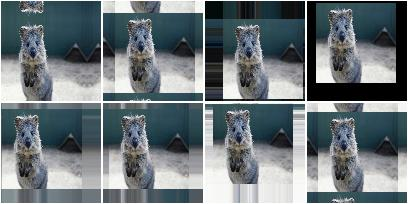Example. Pad images smaller than `100x100` until they reach `100x100`. Analogously, crop images larger than `100x100` until they reach `100x100`. The output images therefore have a fixed size of `100x100`. The input image here has a size of `80x120`, so that the top/bottom sides have to be cropped and the left/right sides have to be padded. Note that the original image was resized to `80x120`, leading to a bit of an distorted appearance.

```aug = iaa.Sequential([
iaa.CropToFixedSize(width=100, height=100)
])
```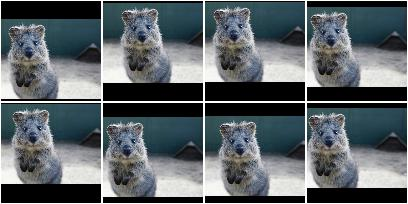## CropToFixedSize¶

Crop images down to a fixed maximum width/height.

If images are already at the maximum width/height or are smaller, they will not be cropped. Note that this also means that images will not be padded if they are below the required width/height.

The augmenter randomly decides per image how to distribute the required cropping amounts over the image axis. E.g. if 2px have to be cropped on the left or right to reach the required width, the augmenter will sometimes remove 2px from the left and 0px from the right, sometimes remove 2px from the right and 0px from the left and sometimes remove 1px from both sides. Set position to `center` to prevent that.

API link: `CropToFixedSize`

Example. For image sides larger than `100` pixels, crop to `100` pixels. Do nothing for the other sides. The cropping amounts are randomly (and uniformly) distributed over the sides of the image. The input image here has a size of `120x120`.

```import imgaug.augmenters as iaa
aug = iaa.CropToFixedSize(width=100, height=100)
```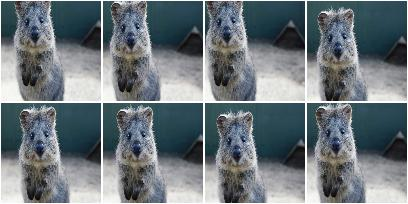Example. For sides larger than `100` pixels, crop to `100` pixels. Do nothing for the other sides. The cropping amounts are always equally distributed over the left/right sides of the image (and analogously for top/bottom). The input image here has a size of `120x120`.

```aug = iaa.CropToFixedSize(width=100, height=100, position="center")
```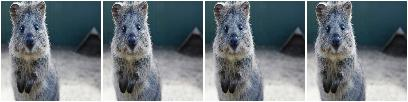Example. Pad images smaller than `100x100` until they reach `100x100`. Analogously, crop images larger than `100x100` until they reach `100x100`. The output images therefore have a fixed size of `100x100`. The input image here has a size of `80x120`, so that the top/bottom sides have to be cropped and the left/right sides have to be padded. Note that the original image was resized to `80x120`, leading to a bit of an distorted appearance.

```aug = iaa.Sequential([
iaa.CropToFixedSize(width=100, height=100)
])
```Pad images until their height/width is a multiple of a value.

API link: `PadToMultiplesOf`

Example. Create an augmenter that pads images to multiples of `10` along the y-axis (i.e. 10, 20, 30, …) and to multiples of `6` along the x-axis (i.e. 6, 12, 18, …). The rows to be padded will be spread randomly over the top and bottom sides (analogous for the left/right sides).

```import imgaug.augmenters as iaa
```

## CropToMultiplesOf¶

Crop images down until their height/width is a multiple of a value.

Note

For a given axis size `A` and multiple `M`, if `A` is in the interval `[0 .. M]`, the axis will not be changed. As a result, this augmenter can still produce axis sizes that are not multiples of the given values.

API link: `CropToMultiplesOf`

Example. Create an augmenter that crops images to multiples of `10` along the y-axis (i.e. 10, 20, 30, …) and to multiples of `6` along the x-axis (i.e. 6, 12, 18, …). The rows to be cropped will be spread randomly over the top and bottom sides (analogous for the left/right sides).

```import imgaug.augmenters as iaa
aug = iaa.CropToMultiplesOf(height_multiple=10, width_multiple=6)
```

## CropToPowersOf¶

Crop images until their height/width is a power of a base.

This augmenter removes pixels from an axis with size `S` leading to the new size `S'` until `S' = B^E` is fulfilled, where `B` is a provided base (e.g. `2`) and `E` is an exponent from the discrete interval `[1 .. inf)`.

Note

This augmenter does nothing for axes with size less than `B^1 = B`. If you have images with `S < B^1`, it is recommended to combine this augmenter with a padding augmenter that pads each axis up to `B`.

API link: `CropToPowersOf`

Example. Create an augmenter that crops each image down to powers of `3` along the y-axis (i.e. 3, 9, 27, …) and powers of `2` along the x-axis (i.e. 2, 4, 8, 16, …). The rows to be cropped will be spread randomly over the top and bottom sides (analogous for the left/right sides).

```import imgaug.augmenters as iaa
aug = iaa.CropToPowersOf(height_base=3, width_base=2)
```

Pad images until their height/width is a power of a base.

This augmenter adds pixels to an axis with size `S` leading to the new size `S'` until `S' = B^E` is fulfilled, where `B` is a provided base (e.g. `2`) and `E` is an exponent from the discrete interval `[1 .. inf)`.

API link: `PadToPowersOf`

Example. Create an augmenter that pads each image to powers of `3` along the y-axis (i.e. 3, 9, 27, …) and powers of `2` along the x-axis (i.e. 2, 4, 8, 16, …). The rows to be padded will be spread randomly over the top and bottom sides (analogous for the left/right sides).

```import imgaug.augmenters as iaa
```

## CropToAspectRatio¶

Crop images until their width/height matches an aspect ratio.

This augmenter removes either rows or columns until the image reaches the desired aspect ratio given in `width / height`. The cropping operation is stopped once the desired aspect ratio is reached or the image side to crop reaches a size of `1`. If any side of the image starts with a size of `0`, the image will not be changed.

API link: `CropToAspectRatio`

Example. Create an augmenter that crops each image until its aspect ratio is as close as possible to `2.0` (i.e. two times as many pixels along the x-axis than the y-axis). The rows to be cropped will be spread randomly over the top and bottom sides (analogous for the left/right sides).

```import imgaug.augmenters as iaa
aug = iaa.CropToAspectRatio(2.0)
```

Pad images until their width/height matches an aspect ratio.

This augmenter adds either rows or columns until the image reaches the desired aspect ratio given in `width / height`.

API link: `PadToAspectRatio`

Example. Create an augmenter that pads each image until its aspect ratio is as close as possible to `2.0` (i.e. two times as many pixels along the x-axis than the y-axis). The rows to be padded will be spread randomly over the top and bottom sides (analogous for the left/right sides).

```import imgaug.augmenters as iaa
```

## CropToSquare¶

Crop images until their width and height are identical.

This is identical to `imgaug.augmenters.size.CropToAspectRatio` with `aspect_ratio=1.0`.

Images with axis sizes of `0` will not be altered.

API link: `CropToSquare`

Example. Create an augmenter that crops each image until its square, i.e. height and width match. The rows to be cropped will be spread randomly over the top and bottom sides (analogous for the left/right sides).

```import imgaug.augmenters as iaa
aug = iaa.CropToSquare()
```

Pad images until their height and width are identical.

This augmenter is identical to `imgaug.augmenters.size.PadToAspectRatio` with `aspect_ratio=1.0`.

API link: `PadToSquare`

Example. Create an augmenter that pads each image until its square, i.e. height and width match. The rows to be padded will be spread randomly over the top and bottom sides (analogous for the left/right sides).

```import imgaug.augmenters as iaa
```

Pad images equally on all sides up to given minimum heights/widths.

This is an alias for `imgaug.augmenters.size.PadToFixedSize` with `position="center"`. It spreads the pad amounts equally over all image sides, while `imgaug.augmenters.size.PadToFixedSize` by defaults spreads them randomly.

API link: `CenterPadToFixedSize`

Example. Create an augmenter that pads images up to `20x30`, with the padded rows added equally on the top and bottom (analogous for the padded columns).

```import imgaug.augmenters as iaa
```

## CenterCropToFixedSize¶

Take a crop from the center of each image.

This is an alias for `imgaug.augmenters.size.CropToFixedSize` with `position="center"`.

Note

If images already have a width and/or height below the provided width and/or height then this augmenter will do nothing for the respective axis. Hence, resulting images can be smaller than the provided axis sizes.

API link: `CenterCropToFixedSize`

Example. Create an augmenter that takes `20x10` sized crops from the center of images:

```import imgaug.augmenters as iaa
crop = iaa.CenterCropToFixedSize(height=20, width=10)
```

## CenterCropToMultiplesOf¶

Crop images equally on all sides until H/W are multiples of given values.

This is the same as `imgaug.augmenters.size.CropToMultiplesOf`, but uses `position="center"` by default, which spreads the crop amounts equally over all image sides, while `imgaug.augmenters.size.CropToMultiplesOf` by default spreads them randomly.

API link: `CenterCropToMultiplesOf`

Example. Create an augmenter that crops images to multiples of `10` along the y-axis (i.e. 10, 20, 30, …) and to multiples of `6` along the x-axis (i.e. 6, 12, 18, …). The rows to be cropped will be spread equally over the top and bottom sides (analogous for the left/right sides).

```import imgaug.augmenters as iaa
aug = iaa.CenterCropToMultiplesOf(height_multiple=10, width_multiple=6)
```

Pad images equally on all sides until H/W are multiples of given values.

This is the same as `imgaug.augmenters.size.PadToMultiplesOf`, but uses `position="center"` by default, which spreads the pad amounts equally over all image sides, while `imgaug.augmenters.size.PadToMultiplesOf` by default spreads them randomly.

API link: `CenterPadToMultiplesOf`

Example. Create an augmenter that pads images to multiples of `10` along the y-axis (i.e. 10, 20, 30, …) and to multiples of `6` along the x-axis (i.e. 6, 12, 18, …). The rows to be padded will be spread equally over the top and bottom sides (analogous for the left/right sides).

```import imgaug.augmenters as iaa
```

## CenterCropToPowersOf¶

Crop images equally on all sides until H/W is a power of a base.

This is the same as `imgaug.augmenters.size.CropToPowersOf`, but uses `position="center"` by default, which spreads the crop amounts equally over all image sides, while `imgaug.augmenters.size.CropToPowersOf` by default spreads them randomly.

API link: `CenterCropToPowersOf`

Example. Create an augmenter that crops each image down to powers of `3` along the y-axis (i.e. 3, 9, 27, …) and powers of `2` along the x-axis (i.e. 2, 4, 8, 16, …). The rows to be cropped will be spread equally over the top and bottom sides (analogous for the left/right sides).

```import imgaug.augmenters as iaa
aug = iaa.CropToPowersOf(height_base=3, width_base=2)
```

Pad images equally on all sides until H/W is a power of a base.

This is the same as `imgaug.augmenters.size.PadToPowersOf`, but uses `position="center"` by default, which spreads the pad amounts equally over all image sides, while `imgaug.augmenters.size.PadToPowersOf` by default spreads them randomly.

API link: `CenterPadToPowersOf`

Example. Create an augmenter that pads each image to powers of `3` along the y-axis (i.e. 3, 9, 27, …) and powers of `2` along the x-axis (i.e. 2, 4, 8, 16, …). The rows to be padded will be spread equally over the top and bottom sides (analogous for the left/right sides).

```import imgaug.augmenters as iaa
```

## CenterCropToAspectRatio¶

Crop images equally on all sides until they reach an aspect ratio.

This is the same as `imgaug.augmenters.size.CropToAspectRatio`, but uses `position="center"` by default, which spreads the crop amounts equally over all image sides, while `imgaug.augmenters.size.CropToAspectRatio` by default spreads them randomly.

API link: `CenterCropToAspectRatio`

Example. Create an augmenter that crops each image until its aspect ratio is as close as possible to `2.0` (i.e. two times as many pixels along the x-axis than the y-axis). The rows to be cropped will be spread equally over the top and bottom sides (analogous for the left/right sides).

```import imgaug.augmenters as iaa
aug = iaa.CenterCropToAspectRatio(2.0)
```

Pad images equally on all sides until H/W matches an aspect ratio.

This is the same as `imgaug.augmenters.size.PadToAspectRatio`, but uses `position="center"` by default, which spreads the pad amounts equally over all image sides, while `imgaug.augmenters.size.PadToAspectRatio` by default spreads them randomly.

API link: `CenterPadToAspectRatio`

Example. Create am augmenter that pads each image until its aspect ratio is as close as possible to `2.0` (i.e. two times as many pixels along the x-axis than the y-axis). The rows to be padded will be spread equally over the top and bottom sides (analogous for the left/right sides).

```import imgaug.augmenters as iaa
```

## CenterCropToSquare¶

Crop images equally on all sides until their height/width are identical.

In contrast to `imgaug.augmenters.size.CropToSquare`, this augmenter always tries to spread the columns/rows to remove equally over both sides of the respective axis to be cropped. `imgaug.augmenters.size.CropToAspectRatio` by default spreads the croppings randomly.

This augmenter is identical to `imgaug.augmenters.size.CropToSquare` with `position="center"`, and thereby the same as `imgaug.augmenters.size.CropToAspectRatio` with `aspect_ratio=1.0, position="center"`.

Images with axis sizes of `0` will not be altered.

API link: `CenterCropToSquare`

Example. Create an augmenter that crops each image until its square, i.e. height and width match. The rows to be cropped will be spread equally over the top and bottom sides (analogous for the left/right sides).

```import imgaug.augmenters as iaa
aug = iaa.CenterCropToSquare()
```

Pad images equally on all sides until their height & width are identical.

This is the same as `imgaug.augmenters.size.PadToSquare`, but uses `position="center"` by default, which spreads the pad amounts equally over all image sides, while `imgaug.augmenters.size.PadToSquare` by default spreads them randomly. This augmenter is thus also identical to `imgaug.augmenters.size.PadToAspectRatio` with `aspect_ratio=1.0, position="center"`.

API link: `CenterPadToSquare`

Example. Create an augmenter that pads each image until its square, i.e. height and width match. The rows to be padded will be spread equally over the top and bottom sides (analogous for the left/right sides).

```import imgaug.augmenters as iaa
```

## KeepSizeByResize¶

Resize images back to their input sizes after applying child augmenters.

Combining this with e.g. a cropping augmenter as the child will lead to images being resized back to the input size after the crop operation was applied. Some augmenters have a `keep_size` argument that achieves the same goal (if set to `True`), though this augmenter offers control over the interpolation mode and which augmentables to resize (images, heatmaps, segmentation maps).

API link: `KeepSizeByResize`

Example. Apply random cropping to input images, then resize them back to their original input sizes. The resizing is done using this augmenter instead of the corresponding internal resizing operation in `Crop`.

```import imgaug.augmenters as iaa
aug = iaa.KeepSizeByResize(
iaa.Crop((20, 40), keep_size=False)
)
```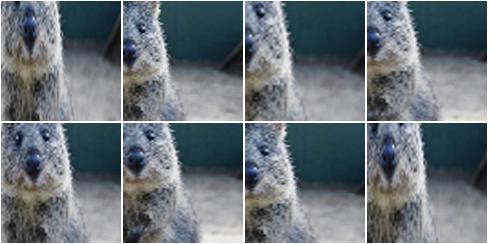Example. Same as in the previous example, but images are now always resized using nearest neighbour interpolation.

```aug = iaa.KeepSizeByResize(
iaa.Crop((20, 40), keep_size=False),
interpolation="nearest"
)
```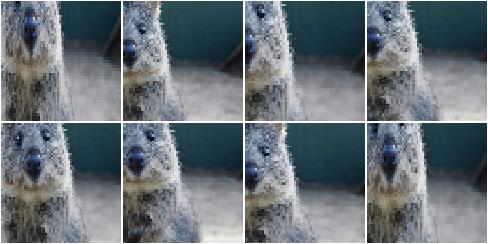Example. Similar to the previous example, but images are now sometimes resized using linear interpolation and sometimes using nearest neighbour interpolation. Heatmaps are resized using the same interpolation as was used for the corresponding image. Segmentation maps are not resized and will therefore remain at their size after cropping.

```aug = iaa.KeepSizeByResize(
iaa.Crop((20, 40), keep_size=False),
interpolation=["nearest", "cubic"],
interpolation_heatmaps=iaa.KeepSizeByResize.SAME_AS_IMAGES,
interpolation_segmaps=iaa.KeepSizeByResize.NO_RESIZE
)
```